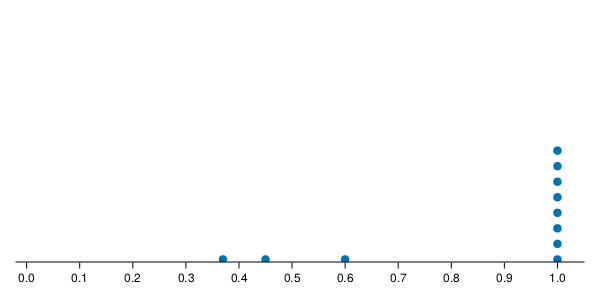# How to move markers in a scatter plot above the x-axis in CairoMakie?

Hi,

I am trying to create a dot plot with CairoMakie
using the `scatter` function. I want the dots to appear above the x-axis spine and decorations. Currently they are being partially hidden as the spine and decorations are being drawn over the markers from the scatter plot. Can this be done? If yes, can someone please suggest a way of doing this. I tried finding something like z-index, z-order from CSS, but could not find it in the docs. Below is my code and the pdf file showing the plot.
Thank you.

``````function draw_dot_plot(df)
size_in = (6,3)
size_pt = 72 .* size_in
fig = Cm.Figure(resolution = size_pt, fontsize = 12)
ax = Cm.Axis(fig[1,1],
bottomspinevisible=true,
topspinevisible=false,
rightspinevisible=false,
leftspinevisible=false,
xticks=0:0.1:1,
xgridvisible=false)
Cm.limits!(-0.02, 1.05, 0.05, 1)
Cm.hideydecorations!(ax)
xvec, yvec = create_dotplot_data(df)
Cm.scatter!(ax, xvec, yvec,
marker = :circle)
Cm.save("./temp/line.pdf", fig, pt_per_unit = 1)
end
``````

PlotTypically it is unusual to have markers extruding out of axes. You can shift the y-axis a little bit so that markers can fully appears inside the plot.

CairoMakie (or any Makie backend) currently doesn’t have the option to disable the rectangular clipping for a `Scene`. What you can do instead is plot into a larger scene, that would be either `ax.blockscene` or `fig.scene`, both of which cover the whole figure and use screen space coordinates. That makes placing dots in data coordinates a bit more complicated because you have to convert the coordinates.

``````f, ax, l = lines(randn(10))
dot_x_dataspace = Observable(3:2:9)
dot_pos_screenspace = lift(dot_x_dataspace, ax.finallimits, ax.scene.px_area) do xs, lims, pxa
points = map(xs) do x
pxa.origin + Point2f(pxa.widths * (x - lims.origin)  / lims.widths, 0)
end
end
sc = scatter!(ax.blockscene, dot_pos_screenspace, markersize = 20)
translate!(sc, 0, 0, 100)
f
``````

Thank you @liuyxpp. I will look at the API for how to shift the y-axis.
Thank you also @jules. I will need to study your solution to understand what it is doing.